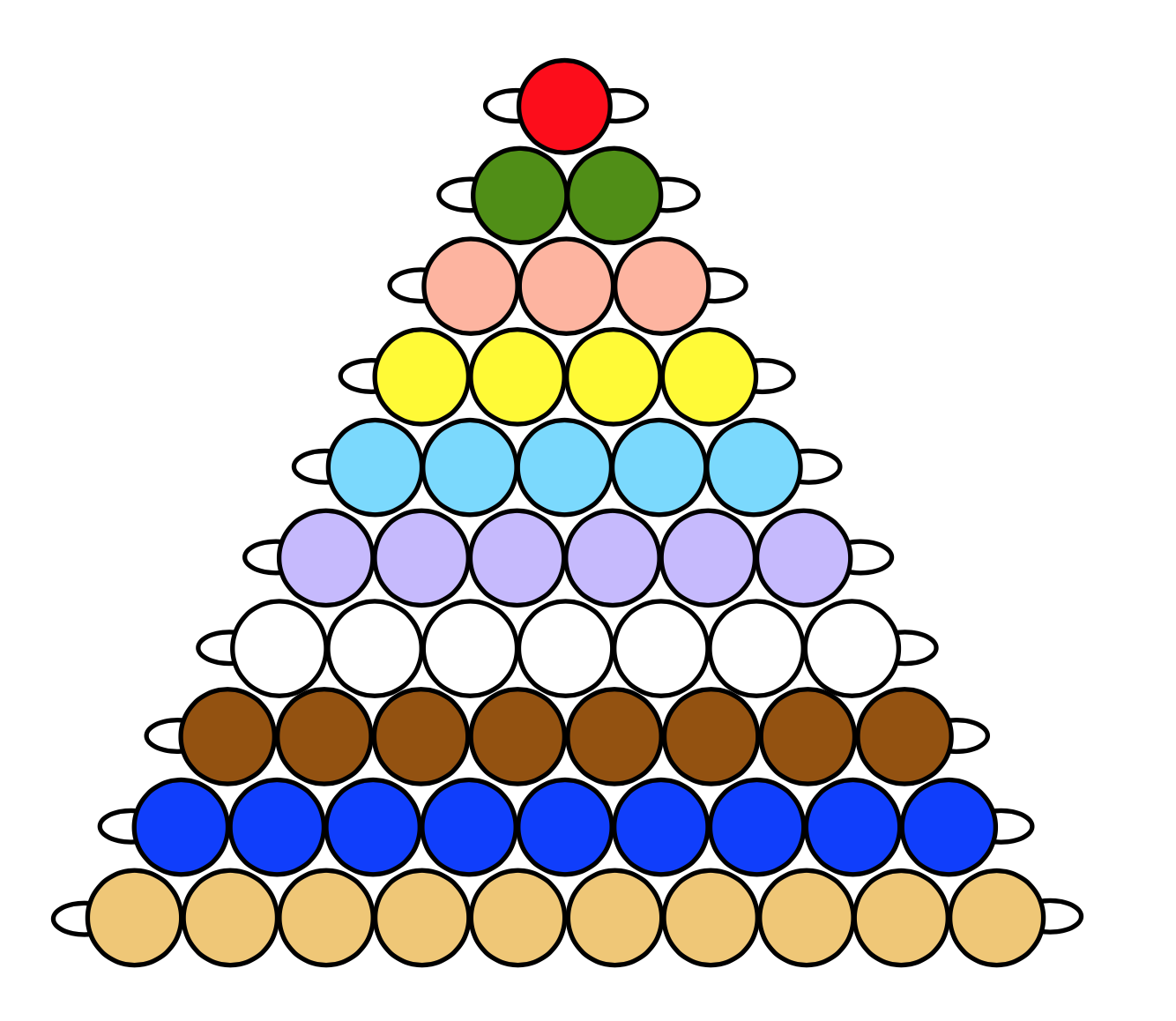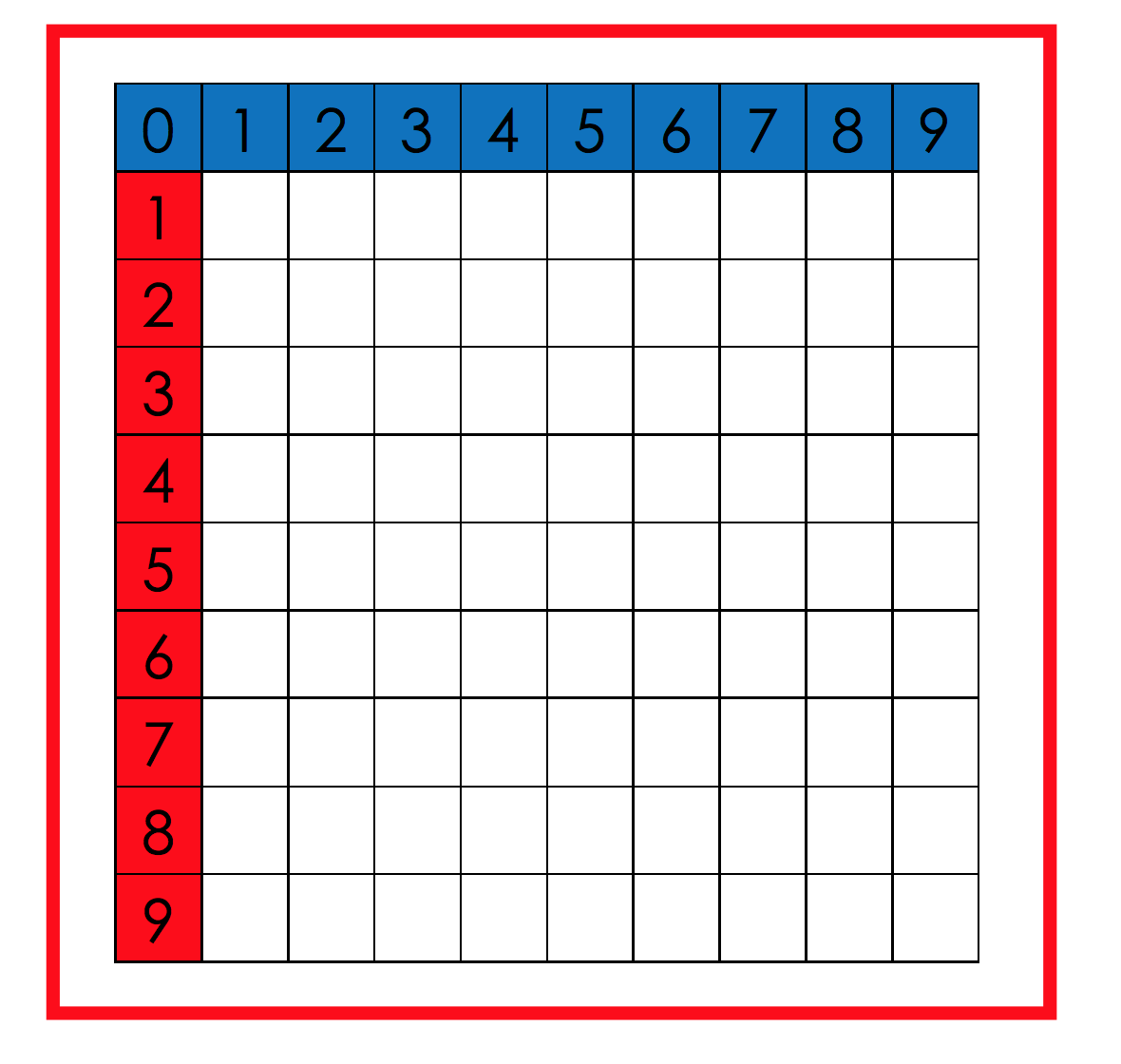# Math

Explore all lessons and activities matching the tag Math## Geometry Cabinet

Discover how two dimensional shapes are introduced.## Geometry-Inspired Activities

Explore shapes, patterns, and other aspects of geometry.## Numbers 1-10

Explore activities for learning numbers 1-10 and zero.## The Decimal System

Explore activities that introduce the decimal system and the four operations.## Counting with Teens and Tens

Explore teens, tens, linear counting and skip counting.## Memorization

Explore activities that help children memorize addition, subtraction, multiplication, and division math facts.